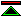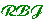# Representing Computable Reals

First I'm going to try state the problem then I'm going to say what I know about how to tell whether a representation shapes up, and then I'm going to run through some candidates that are not suitable and some candidates that are suitable.

## The Problem

There are lots of different problems you can work on in this area. There is one problem which I think is particularly important.

In brief I suggest that we should be looking for two features in a representation:

1. The set of reals represented is the computable reals.
2. Over the representation those operations are effective (i.e. computable) which we would expect to be effective.
I suspect that this should be the same set of operations as are shown to be constructive in
Bishop85. However, for present purposes its sufficient to insist on a very basic minimum. Just addition will do for our discussion.

To characterise the problem, first I talk about the numbers, and then just a little about the kinds of operations we should be able to perform over them.

The numbers are easy to fix, they are the computable reals. A good enough definition of these is:

Those real numbers for which there exists a turing machine which writes the binary expansion of the number onto its tape.

I think this is a good definition, though more definite than it need be. It doesn't matter what base (>1) you use. I believe that this definition determines an important subset of the reals.

Here's another definition:

The computable reals are the limits of effectively enumerable convergent sequences of rationals.

## Criteria

First you have to represent enough of the real numbers. Representing the computable reals is good enough. Normally some infinite sequence of finite structures (e.g. rationals) is the conceptual basis for the representation, and it is understood that the sequences actually representable will be the computable sequences.

The range of functions over reals which are computable depends upon the details however. To maximise the utility in this sense futher criteria are appropriate. This was known by Turing as early as 1937, but the earliest approach to describing the necessary conditions I have found is in [Wiedmer76]. More recently [Weihrauch95b] (and probably his earlier publications) uses the term admissible representation to refer to representation schemes which meet the extra criteria. Assuming that the representation involves infinite sequences of finite structures, each finite prefix gives an approximation to a real number and determines a the set of reals having representatives which begin with the sequence in question. These sets of reals determine a topology on the set of real representations, and enable us to talk about continuity of the abstraction function from these representatives to the real numbers which they represent.

A system of representation for reals is admissible if this abstraction function is continuous.©created 1995/9/3 modified 1996/2/12